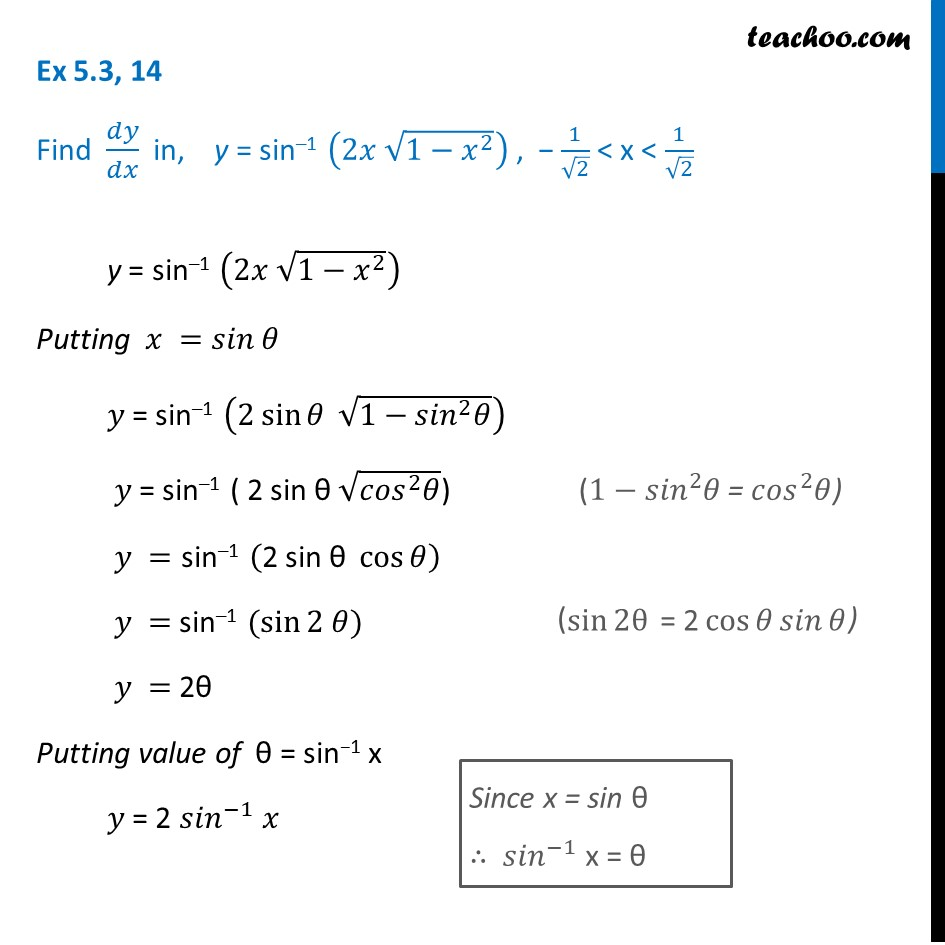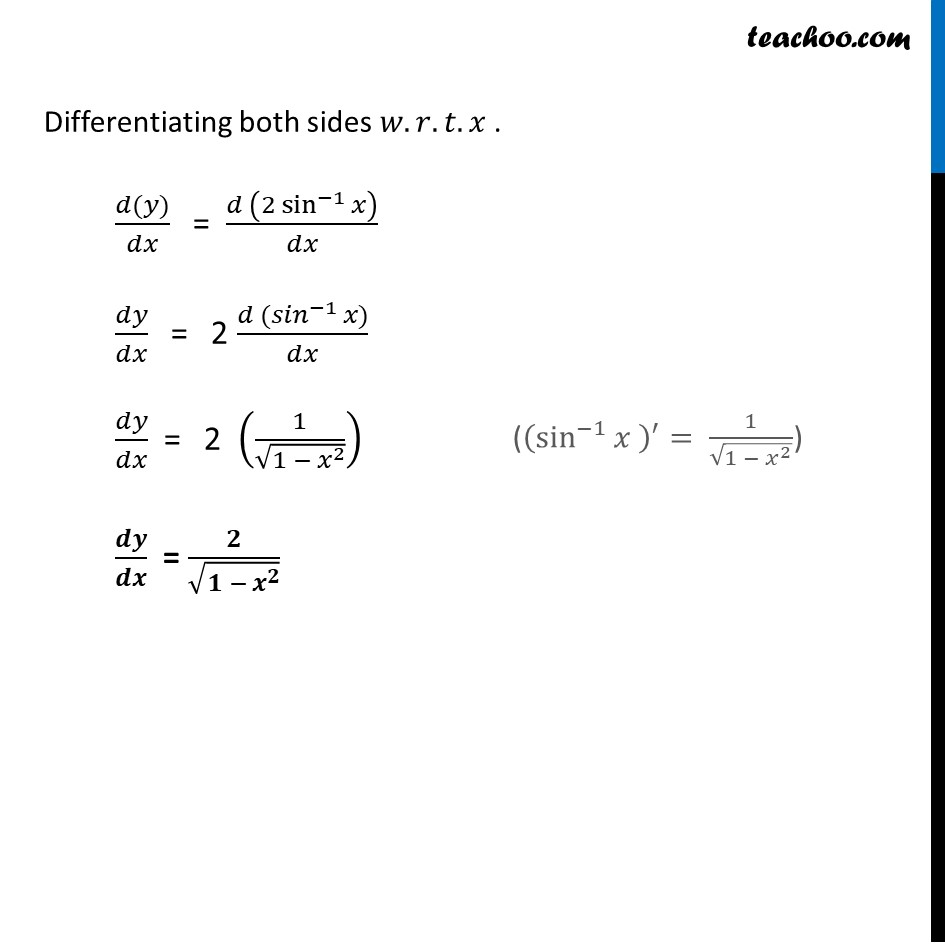Ex 5.3

Chapter 5 Class 12 Continuity and Differentiability
Serial order wiseLearn in your speed, with individual attention - Teachoo Maths 1-on-1 Class

### Transcript

Ex 5.3, 14 Find 𝑑𝑦/𝑑𝑥 in, y = sin–1 (2𝑥 √(1−𝑥^2 )) , − 1/√2 < x < 1/√2 y = sin–1 (2𝑥 √(1−𝑥^2 )) Putting 𝑥 =𝑠𝑖𝑛⁡𝜃 𝑦 = sin–1 (2 sin⁡𝜃 √(1−〖𝑠𝑖𝑛〗^2 𝜃)) 𝑦 = sin–1 ( 2 sin θ √(〖𝑐𝑜𝑠〗^2 𝜃)) 𝑦 ="sin–1 " (〖"2 sin θ" 〗⁡cos⁡𝜃 ) 𝑦 = sin–1 (sin⁡〖2 𝜃)〗 𝑦 = 2θ Putting value of θ = sin−1 x 𝑦 = 2 〖𝑠𝑖𝑛〗^(−1) 𝑥 Since x = sin θ ∴ 〖𝑠𝑖𝑛〗^(−1) x = θ Differentiating both sides 𝑤.𝑟.𝑡.𝑥 . (𝑑(𝑦))/𝑑𝑥 = (𝑑 (〖2 sin^(−1)〗⁡𝑥 ))/𝑑𝑥 𝑑𝑦/𝑑𝑥 = 2 (𝑑〖 (𝑠𝑖𝑛〗^(−1) 𝑥))/𝑑𝑥 𝑑𝑦/𝑑𝑥 = 2 (1/√(1 −〖 𝑥〗^2 )) 𝒅𝒚/𝒅𝒙 = 𝟐/√(𝟏 − 𝒙^𝟐 ) ((sin^(−1)⁡𝑥 )^′= 1/√(1 − 𝑥^2 ))